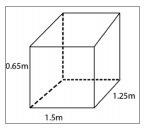Ncert solutionsOur top 5% students will be awarded a special scholarship to Lido.
Set your child up for success with Lido, book a class today!

# Question 1

A plastic box 1.5 m long, 1.25 m wide, and 65 cm deep, is to be made. It is to be open at the top.

Ignoring the thickness of the plastic sheet, determine:

(i) The area of the sheet required for making the box.

(ii) The cost of the sheet for it, if a sheet measuring 1 m^2 costs Rs. 20.

• Solution

• TranscriptGiven: length (l) of box = 1.5m

Breadth (b) of box = 1.25 m

Depth (h) of box = 0.65m

(i) The box is to be open at the top

Area of sheet required.

\begin{array}{l} =2 \mathrm{ln}+2 \mathrm{bh}+\mathrm{lb} \\ =[2 \times 1.5 \times 0.65+2 \times 1.25 \times 0.65+1.5 \times 1.25] \mathrm{m}^{2} \\ =(1.95+1.625+1.875) \mathrm{m}^{2}=5.45 \mathrm{m}^{2} \end{array}

(ii) Cost of sheet per m^2 area = Rs.20.

Cost of sheet of 5.45 m^2 area =Rs (5.45×20)

= Rs.109.

"hello students welcome to lido q a video session i am seth your math tutor and question for today is a plastic box 1.5 meter long 1.25 meter wide and 65 centimeter d is to be made it is to be opened at the top ignoring the thickness of the plastic sheet determine first the area of the sheet that is required for making the box second the cost of the sheet for it if a sheet measuring one meter square cost rupees 20 so let us see first of all what is given given is the length of the plastic box length of plastic box l will be equal to 1.5 meter breadth b will be equal to 1.25 meter and height h will be equal to 0.65 meter and that is given here centimeter which is given in as a height is converted directly into meter as 0.65 meter now let us find the first one the area of the sheet required for making the box so first one it can be found by first of all the box is to be open at the top and hence the area of the sheet required will be two into length into breath plus two into breath into height and one end is not there so we will take one time length into breadth let us write it down so area of the sheet required will be equal to 2 into length into breath or we can write like this 2 into length into height plus 2 into breath into height plus 2 into length into breath but here one end is open so we don't have to write 2 into length into breath we just have to write 1 times length into breath so it is understood now that the box is to be open at the top right down here in the bracket so finally we can substitute the respective values 2 into 1.5 into 0.65 plus 2 into 1.25 into 0.65 plus 1.5 into 1.25 and that is meter square so we can finally write here one point nine five plus one point six six two five plus 1.875 meter square will be finally equal to 5.45 meter square that is our area of the sheet required denoted by a so first part is solved let us see the second part in the second part you have been given that the cost of the sheet for it if a sheet measuring 1 meter square cost rupees 20 we have found the area over here at below and that is 5.45 meter square now let us find the cost of sheet for it so cost of sheet per meter square is given so this is the second part so how much will be the cost of sheet 5.45 meter square will be equal to 20 into 5.45 and hence we can finally solve it over here like cost will be equal to that is required cost 20 into 5.45 and that is equal to 109 rupees so area of sheet required is 5.45 which we founded previously meter square and cost of sheet is equal to 109 rupees so these are our answers if you have any query you can post it in the comment section and subscribe to lido for more such q a thank you for watching "

Our top 5% students will be awarded a special scholarship to Lido.Lido

Courses

Teachers

Book a Demo with us

Syllabus

Science
English
Coding

Terms & Policies

NCERT Question Bank

Maths
Science

Selina Question Bank

Maths
Physics
Biology

Allied Question Bank

Chemistry